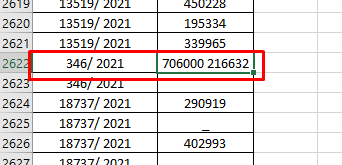# Excel Automation for duplicate values

Dear Team,

In excel file, there were two columns. One with many duplicate values and other with the respective amount. I need to merge the one value to sum the amount. Please help me with this.

Can you share the sample Input and Output excel file?

This is the file Sir

``````(From d In DtBuild.AsEnumerable
Group d By k=d("Column 1").toString.Trim Into grp = Group
Let nj = grp.Sum(Function (x) CDbl(x("Column 2").toString.Trim))
Let ra = New Object(){k,nj}
``````

Regards
Gokul

I tried Sir, but its not working.
in the duplicate values, need to merge the value and sum the amount

Your excel file is not good, It has `-` and some value like in the screenshot.Try with this expression

``````(From d In DtBuild.AsEnumerable
Group d By k=d("W.P.No").toString.Trim Into grp = Group
Let nj = (From d In grp.AsEnumerable Where Not (isNothing(d(1)) OrElse String.IsNullorEmpty(d(1).toString.Trim) OrElse d(1).tostring.Contains("-")) Select v = CDbl(d(1).toString.Trim)).Sum(Function (x) x)
Let ra = New Object(){k,nj}
``````

Regards
Gokul

Yes sir,
Thats the issue. Is there any other possible solution. please let me know

``````(From d In DtBuild.AsEnumerable
Group d By k=d("W.P.No").toString.Trim Into grp = Group
Let nj = (From d In grp.AsEnumerable Where Not (isNothing(d(1)) OrElse String.IsNullorEmpty(d(1).toString.Trim) OrElse d(1).tostring.Contains("-") OrElse String.IsNullOrWhiteSpace(d(1).tostring.trim)) Select v = CDbl(System.Text.RegularExpressions.Regex.Replace(d(1).Tostring.Trim," ","").Tostring)).Sum(Function (x) x)
Let ra = New Object(){k,nj}